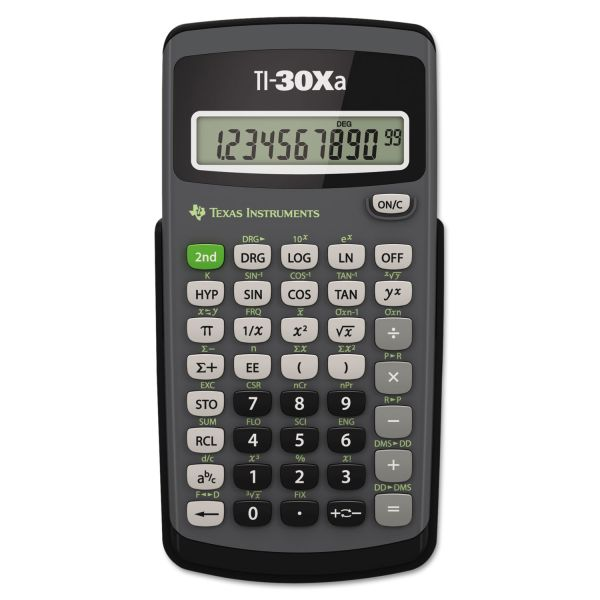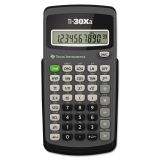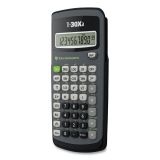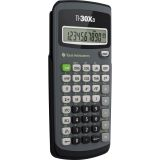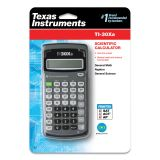Top Seller

Texas Instruments TI-30Xa Scientific Calculator, 10-Digit LCD

Product Number: TEXTI30XA
• Performs basic scientific and trigonometric functions.
• Ideal for general math, pre-algebra, algebra 1 and 2, trigonometry and biology.
• Features conversions and fraction calculations.
More details
\$9.65
Free Shipping Orders Over \$45+ Estimated Delivery Thu 1/20 (3 business days) Thu 1/20 (4 business days)
Favorite
Compare
Request Quote

Product Description

Texas Instruments TI-30Xa Scientific Calculator, 10-Digit LCD - 10-Digit Scientific Calculator is ideal for general math, pre-algebra, algebra 1 and 2, trigonometry and biology. Performs trigonometric functions, logarithms, roots, powers, reciprocals, and factorials. One-variable statistics include results for mean and standard deviation. Calculator also adds, subtracts, multiplies, and divides fractions entered in traditional numerator/denominator format. Conversions include fractions/decimals, degrees/radians/grads, DMS/decimal/degrees, and polar/rectangular. Battery-powered calculator includes slide case.

• Performs basic scientific and trigonometric functions.
• Ideal for general math, pre-algebra, algebra 1 and 2, trigonometry and biology.
• Features conversions and fraction calculations.Guides & Information
Related Articles

Product Specifications

• +/- Switch Key
Yes
1-Year Limited
• Amortization
No
• Assembly Required
No
• Backspace Key
Yes
• Base Number Calculations
No
• Bond Calculations
No
• Brand Name
Texas Instruments
• Case
Hard Case
• Color
Black
• Complex Number Calculations
No
• Confidence Interval Calculating
No
• Cost/Sell/Margin
No
• Country of Origin
CN
• Currency Exchange Function
No
• Date Calculations
No
• Decimal Function
No
• Depreciation Calculations
No
• Depth
0.8"
• Dimensions
6 in. X 3 1/10 in. X 0 4/5 in.
• Display Characters Height
8.3 mm
• Display Characters x Display Lines
10 x 1
• Display Notation
Numeric
• Display Type(s)
LCD
• Double Zero Key
No
• Entry Logic
Algebraic
• Equation Editor
No
• Fraction/Decimal Conversions
Yes
• Fraction Calculations
Yes
• Global Product Type
Calculators-Scientific
• Grand Total Key
No
• Height
6"
• Hyperbolic Functions
No
• Hypothesis Testing
No
• Interest Rate Conversion
No
• Item Count Function
No
• Key Size
Medium
• Levels of Parentheses
10
• Linear Regression
No
• Loan Calculation
No
• Logical (Boolean) Operations
No
• Manufacturer
Texas Instruments, Inc
• Manufacturer Part Number
TI30XA
www.ti.com
• Markup/Down Key
No
• Matrices
No
• Memory
1
• Metric Conversion
No
• Model
TI-30Xa
• Number of Batteries
2
• Number of Display Digits
10
• Number of Functions
120
• Package Contents
TI30XA Scientific Calculator Slide Case
• Packaging
1
No
• Percent Key(s)
No
• Polar-Rectangular Conversion
Yes
• Post-Consumer Recycled Content Percent
0%
• Power Source
Battery
• Power Source(s)
Battery
• Pre-Consumer Recycled Content Percent
0%
• Probability (Random Number)
No
• Product Model
TI30XA
• Product Name
TI-30XA Student Scientific Calculator
• Product Type
Scientific Calculator
• Recycled
No
• Refurbished
N
• Replacement Batteries
Silver Oxide
• Screen Size
2.31 in.
• Simultaneous Equations
No
• Size
3.13 x 6
• Square Root Key
Yes
• Tax Calculation
No
• Time-Value-of-Money
No
• Time/Date
No
• Total Recycled Content Percent
0%
• Trig/Log Functions
Yes
• Variable Regression
No
• Variable Statistics
One
• Width
3.1"